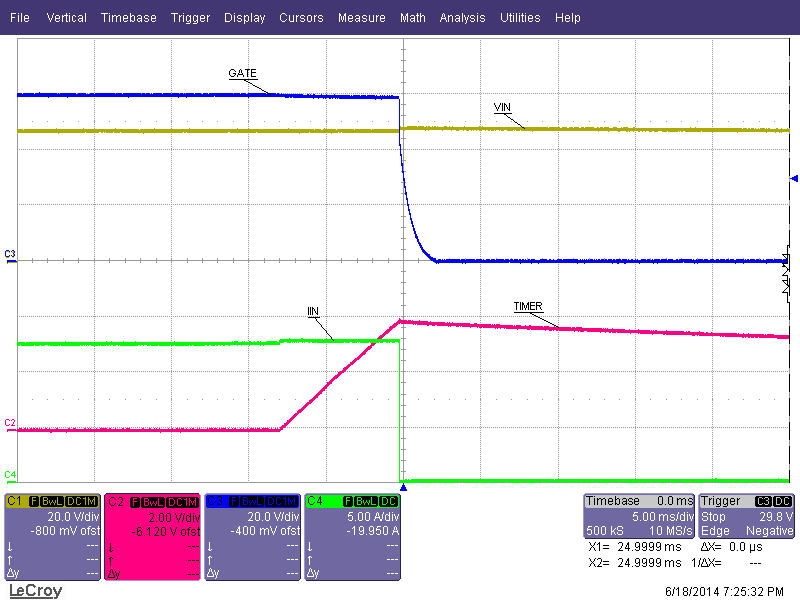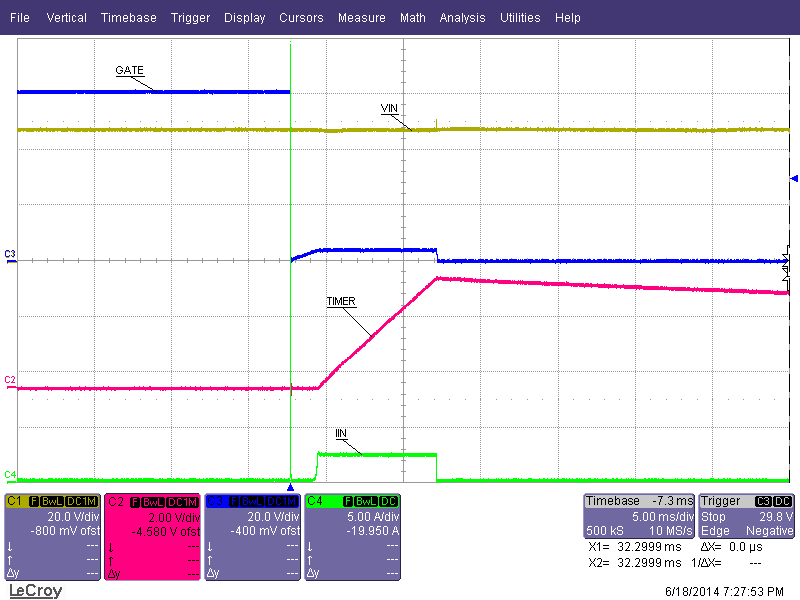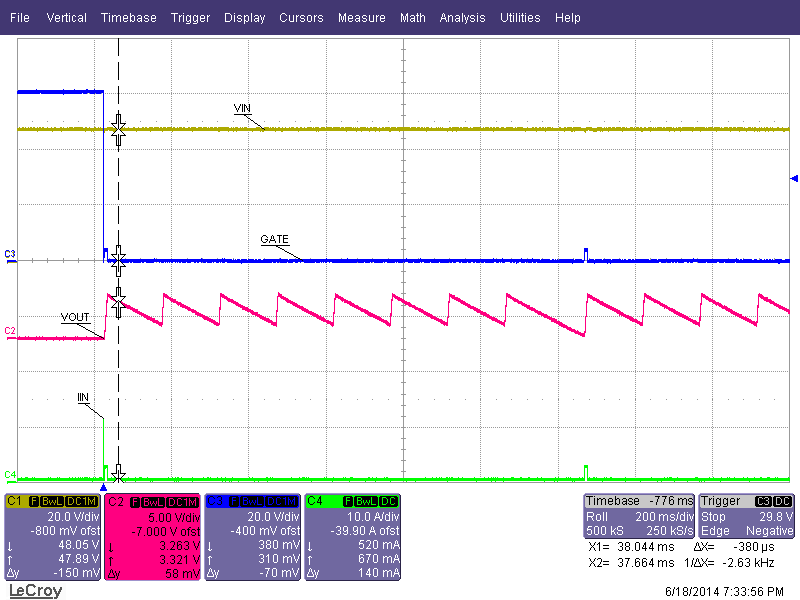SNVS655I June   2011  – January 2016

PRODUCTION DATA.

1. Features
2. Applications
3. Description
4. Revision History
5. Device Comparison Table
6. Pin Configuration and Functions
7. Specifications
8. Detailed Description
1. 8.1 Overview
2. 8.2 Functional Block Diagram
3. 8.3 Feature Description
4. 8.4 Device Functional Modes
5. 8.5 Programming
9. Application and Implementation
1. 9.1 Application Information
2. 9.2 Typical Application
1. 9.2.1 48-V, 10-A PMBus Hotswap Design
1. 9.2.1.1 Design Requirements
2. 9.2.1.2 Detailed Design-In Procedure
3. 9.2.1.3 Application Curves
10. 10Power Supply Recommendations
11. 11Layout
12. 12Device and Documentation Support
13. 13Mechanical, Packaging, and Orderable Information

• PWP|28
• PWP|28

## 9 Application and Implementation

### 9.1 Application Information

The LM5066 is a hotswap with a PMBus interface that provides current, voltage, power, and status information to the host. As a hotswap, it is used to manage inrush current and protect in case of faults.

When designing a hotswap, three key scenarios should be considered:

• Start-up
• Output of a hotswap is shorted to ground when the hotswap is on. This is often referred to as a hot-short.
• Powering-up a board when the output and ground are shorted. This is usually called a start-into-short.

All of these scenarios place a lot of stress on the hotswap MOSFET and take special care when designing the hotswap circuit to keep the MOSFET within its SOA. Detailed design examples are provided in the following sections. Solving all of the equations by hand is cumbersome and can result in errors. Instead, TI recommends to use the LM5066 Design Calculator provided on the product page.

### 9.2 Typical Application

#### 9.2.1 48-V, 10-A PMBus Hotswap Design

This section describes the design procedure for a 48-V, 10-A PMBUS hotswap design.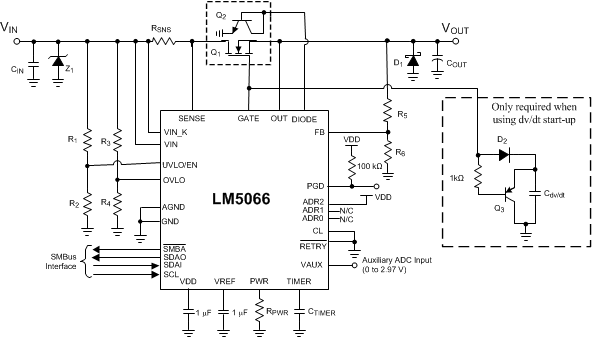Figure 28. Typical Application Circuit

#### 9.2.1.1 Design Requirements

Table 46 summarizes the design parameters that must be known before designing a hotswap circuit. When charging the output capacitor through the hotswap MOSFET, the FET’s total energy dissipation equals the total energy stored in the output capacitor (1 / 2CV2). Thus, both the input voltage and output capacitance determine the stress experienced by the MOSFET. The maximum load current drives the current limit and sense resistor selection. In addition, the maximum load current, maximum ambient temperature, and thermal properties of the PCB (RθCA) drive the selection of the MOSFET RDSON and the number of MOSFETs used. RθCA is a strong function of the layout and the amount of copper that is connected to the drain of the MOSFET. Note that the drain is not electrically connected to the ground plane, and thus the ground plane cannot be used to help with heat dissipation. This design example uses RθCA = 30°C/W, which is similar to the LM5066 EVM. It is a good practice to measure the RθCA of a given design after the physical PCBs are available.

Finally, it is important to understand what test conditions the hotswap needs to pass. In general, a hotswap is designed to pass both a hot-short and a start into a short, which are described in the previous section. Also, TI recommends to keep the load OFF until the hotswap is fully powered-up. Starting the load early causes unnecessary stress on the MOSFET and could lead to MOSFET failures or a failure to start-up.

### Table 46. Design Parameters

PARAMETER EXAMPLE VALUE
Input voltage range 40 to 60 V
Maximum output capacitance of the hotswap 220 µF
Maximum ambient temperature 85°C
MOSFET RθCA (function of layout) 30°C/W
Pass hot-short on output? Yes
Pass a start into short? Yes
Is the load off until PG asserted? Yes
Can a hot board be plugged back in? Yes

#### 9.2.1.2.1 Select RSNS and CL Setting

LM5066 can be used with a VCL of 26 or 50 mV. Using the 26-mV threshold results in a lower RSNS and lower I2R losses, but using the 50-mV threshold would result in better current and power monitoring accuracy along with a lower minimum power limit . The 26-mV option is selected for this design by connecting the CL pin directly to VDD. TI recommends to target a current limit that is at least 10% above the maximum load current to account for the tolerance of the LM5066 current limit. Targeting a current limit of 11 A, the sense resistor can be computed as follows:

Equation 5.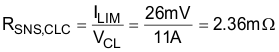Typically, sense resistors are only available in discrete values. If a precise current limit is desired, a sense resistor along with a resistor divider can be used as shown in Figure 29.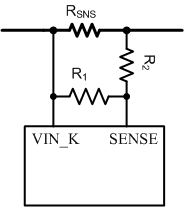Figure 29. SENSE Resistor Divider

The next larger available sense resistor should be chosen (3 mΩ in this case). The ratio of R1 and R2 can be computed as follows:

Equation 6.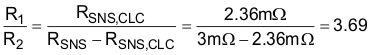Note that the SENSE pin pulls 25 μA of current, which creates an offset across R2. TI recommends to keep R2 below 10 Ω to reduce the offset that this introduces. In addition, the 1% resistors add to the current monitoring error. Finally, if the resistor divider approach is used, the user should compute the effective sense resistance (RSNS,EFF) using Equation 7 and use that in all equations instead of RSNS.

Equation 7.Note that for many applications, a precise current limit may not be required. In that case, it is simpler to pick the next smaller available sense resistor. For this application, a 2-mΩ resistor can be used for a 13-A current limit.

#### 9.2.1.2.2 Selecting the Hotswap FETs

It is critical to select the correct MOSFET for a hotswap design. The device must meet the following requirements:

• The VDS rating should be sufficient to handle the maximum system voltage along with any ringing caused by transients. For most 48-V systems, a 100-V FET is a good choice.
• The SOA of the FET should be sufficient to handle all usage cases: start-up, hot-short, and start into short.
• RDSON should be sufficiently low to maintain the junction and case temperature below the maximum rating of the FET. In fact, TI recommends to keep the steady-state FET temperature below 125°C to allow margin to handle transients.
• Maximum continuous current rating should be above the maximum load current and the pulsed-drain current must be greater than the current threshold of the circuit breaker. Most MOSFETs that pass the first three requirements also pass these two.
• A VGS rating of ±20 V is required because the LM5066 can pull up the gate as high as 16 V above source.

For this design, the PSMN4R8-100BSE was selected for its low RDSON and superior SOA. After selecting the MOSFET, the maximum steady-state case temperature can be computed as follows:

Equation 8.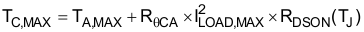Note that the RDSON is a strong function of junction temperature, which for most D2PACK MOSFETs is very close to the case temperature. A few iterations of the previous equations may be necessary to converge on the final RDSON and TC,MAX value. According to the PSMN4R8-100BSE data sheet, it's RDSON doubles at 110°C. Equation 9 uses this RDSON value to compute the TC,MAX. Note that the computed TC,MAX is close to the junction temperature assumed for RDSON. Thus, no further iterations are necessary.

Equation 9.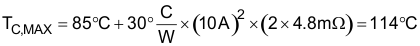#### 9.2.1.2.3 Select Power Limit

In general, a lower power limit setting is preferred to reduce the stress on the MOSFET. However, when the LM5066 is set to a very-low power limit setting, it has to regulate the FET current and hence the voltage across the sense resistor (VSNS) to a very-low value. VSNS can be computed as shown in Equation 10.

Equation 10.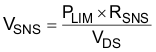To avoid significant degradation of the power limiting, TI does not recommend a VSNS of less than 4 mV. Based on this requirement, the minimum allowed power limit can be computed as follows:

Equation 11.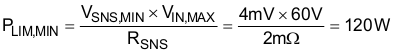In most applications, the power limit can be set to PLIM,MIN using Equation 12. Note that the PLIM of the LM5066 will have some variations vs VDS of the MOSFET due to a 1.5-mV systematic offset. Equation 12 sets RPWR to make the actual power limit equal the programmed power limit at VIN = VIN,MAX and VOUT = 0 V.

Equation 12.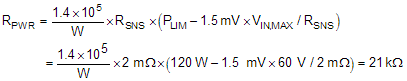The closest available resistor should be selected. In this case, a 21-kΩ resistor was chosen.

#### 9.2.1.2.4 Set Fault Timer

The fault timer runs when the hotswap is in power limit or current limit, which is the case during start-up. Thus, the timer has to be sized large enough to prevent a time-out during start-up. If the part starts directly into current limit (ILIM × VDS < PLIM), the maximum start time can be computed with Equation 13.

Equation 13.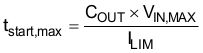For most designs (including this example), ILIM × VDS > PLIM, so the hotswap starts in power limit and transitions into current limit. In that case, the maximum start time can be computed as in Equation 14.

Equation 14.Note that the above start-time is based on typical current limit and power limit values. To ensure that the timer never times out during start-up, TI recommends to set the fault time (tflt) to be 2 × tstart,max or 6.76 ms. This accounts for the variation in power limit, timer current, and timer capacitance. Thus, CTIMER can be computed as follows:

Equation 15.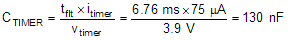The next largest standards capacitor value for CTIMER is chosen as 150 nF. After CTIMER is chosen, the actual programmed fault time can be computed as follows:

Equation 16.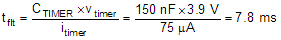#### 9.2.1.2.5 Check MOSFET SOA

When the power limit and fault timer are chosen, it is critical to check that the FET stays within its SOA during all test conditions. During a hot-short the circuit breaker trips and the LM5066 restarts into power limit until the timer runs out. In the worst case, the MOSFET’s VDS equals VIN,MAX, IDS equals PLIM / VIN,MAX and the stress event lasts for tflt. For this design example, the MOSFET has 60 V, 2 A across it for 7.8 ms.

Based on the SOA of the PSMN4R8-100BSE, it can handle 60 V, 30 A for 1 ms and it can handle 60 V, 6 A for 10 ms. For 7.8 ms, the SOA can be extrapolated by approximating SOA versus time as a power function as shown below:

Equation 17.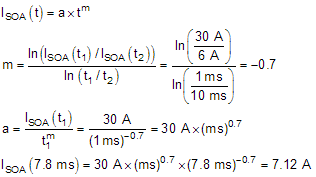Note that the SOA of a MOSFET is specified at a case temperature of 25°C, while the case temperature can be much hotter during a hot-short. The SOA should be de-rated based on TC,MAX using Equation 18:

Equation 18.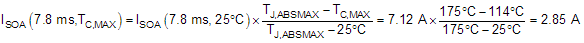Based on this calculation, the MOSFET can handle 2.85 A, 60 V for 7.8 ms at elevated case temperature, but is only required to handle 2 A during a hot-short. Thus, there is good margin and the design is robust. In general, TI recommends that the MOSFET can handle 1.3× more than what is required during a hot-short. This provides margin to account for the variance of the power limit and fault time.

#### 9.2.1.2.6 Set UVLO and OVLO Thresholds

By programming the UVLO and OVLO thresholds, the LM5066 enables the series-pass device (Q1) when the input supply voltage (VIN) is within the desired operational range. If VIN is below the UVLO threshold or above the OVLO threshold, Q1 is switched off, denying power to the load. Hysteresis is provided for each threshold.

#### 9.2.1.2.6.1 Option A

The configuration shown in Figure 30 requires three resistors (R1 to R3) to set the thresholds.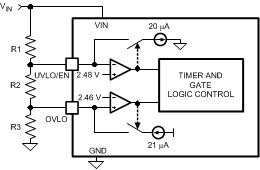Figure 30. UVLO And OVLO Thresholds Set By R1-R3

The procedure to calculate the resistor values is as follows:

• Choose the upper UVLO threshold (VUVH) and the lower UVLO threshold (VUVL).
• Choose the upper OVLO threshold (VOVH).
• The lower OVLO threshold (VOVL) cannot be chosen in advance in this case, but is determined after the values for R1 to R3 are determined. If VOVL must be accurately defined in addition to the other three thresholds, see Option B. The resistors are calculated as follows:
Equation 19.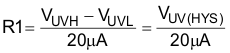Equation 20.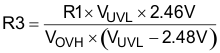Equation 21.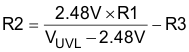The lower OVLO threshold is calculated from:

Equation 22.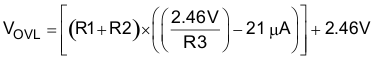When the R1 to R3 resistor values are known, the threshold voltages and hysteresis are calculated from the following:

Equation 23.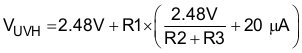Equation 24.Equation 25.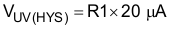Equation 26.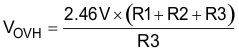Equation 27.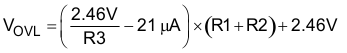Equation 28.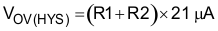#### 9.2.1.2.6.2 Option B

If all four thresholds must be accurately defined, the configuration in Figure 31 can be used.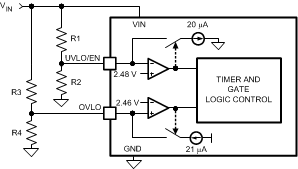Figure 31. Programming the Four Thresholds

The four resistor values are calculated as follows:

• Choose the upper and lower UVLO thresholds (VUVH) and (VUVL).
• Equation 29.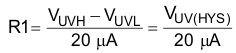Equation 30.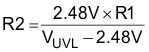• Choose the upper and lower OVLO threshold (VOVH) and (VOVL).
• Equation 31.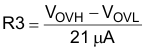Equation 32.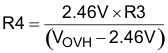• When the R1 to R4 resistor values are known, the threshold voltages and hysteresis are calculated from the following:
Equation 33.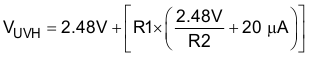Equation 34.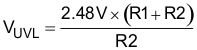Equation 35.Equation 36.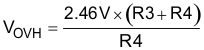Equation 37.#### 9.2.1.2.6.3 Option C

The minimum UVLO level is obtained by connecting the UVLO/EN pin to VIN as shown in Figure 32. Q1 is switched on when the VIN voltage reaches the POREN threshold (≊8.6 V). The OVLO thresholds are set using R3, R4. Their values are calculated using the procedure in Option B.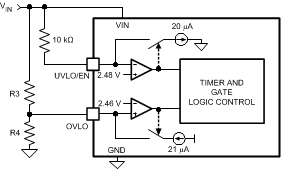Figure 32. UVLO = POREN

#### 9.2.1.2.6.4 Option D

The OVLO function can be disabled by grounding the OVLO pin. The UVLO thresholds are set as described in Option B or Option C.

For this design example, option B was used and the following options were targeted: VUVH = 38 V, VUVL = 35 V, VOVH = 65 V, and VOVL = 63 V. The VUVH and VOVL were chosen to be 5% below or above the input voltage range of 40 to 60 V to allow for some tolerance in the thresholds of the part. R1, R2, R3, and R4 are computed using the following equations:

Equation 38.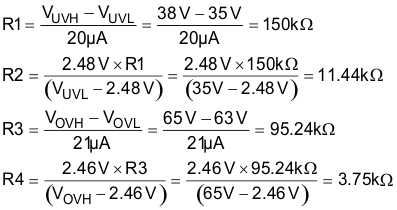Nearest available 1% resistors should be chosen. Set R1 = 150 kΩ, R2 = 11.5 kΩ, R3 = 95.3 kΩ, and R4 = 3.74 kΩ.

#### 9.2.1.2.7 Power Good Pin

The Power Good indicator pin (PGD) is connected to the drain of an internal N-channel MOSFET capable of sustaining 80 V in the off-state and transients up to 100 V. An external pullup resistor is required at PGD to an appropriate voltage to indicate the status to downstream circuitry. The off-state voltage at the PGD pin can be higher or lower than the voltages at VIN and OUT. PGD is switched high when the voltage at the FB pin exceeds the PGD threshold voltage. Typically, the output voltage threshold is set with a resistor divider from output to feedback, although the monitored voltage need not be the output voltage. Any other voltage can be monitored as long as the voltage at the FB pin does not exceed its maximum rating. Referring to the Functional Block Diagram, when the voltage at the FB pin is below its threshold, the 20-µA current source at FB is disabled. As the output voltage increases, taking FB above its threshold, the current source is enabled, sourcing current out of the pin, raising the voltage at FB to provide threshold hysteresis. The PGD output is forced low when either the UVLO/EN pin is below its threshold or the OVLO pin is above its threshold. The status of the PGD pin can be read through the PMBus interface in either the STATUS_WORD (79h) or DIAGNOSTIC_WORD (E1h) registers.

When the voltage at the FB pin increases above its threshold, the internal pulldown acting on the PGD pin is disabled allowing PGD to rise to VPGD through the pullup resistor, RPG, as shown in Figure 34. The pullup voltage (VPGD) can be as high as 80 V, and can be higher or lower than the voltages at VIN and OUT. VDD is a convenient choice for VPGD as it allows interface to low voltage logic and avoids glitching on PGD during power-up. If a delay is required at PGD, suggested circuits are shown in Figure 35. In Figure 35(A), capacitor CPG adds delay to the rising edge, but not to the falling edge. In Figure 35(B), the rising edge is delayed by RPG1 + RPG2 and CPG, while the falling edge is delayed a lesser amount by RPG2 and CPG. Adding a diode across RPG2 (Figure 35(C)) allows for equal delays at the two edges, or a short delay at the rising edge and a long delay at the falling edge.Figure 33. Programming the PGD Threshold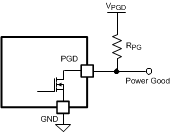Figure 34. Power Good Output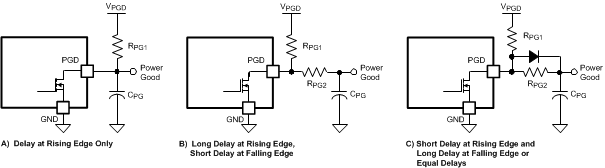Figure 35. Adding Delay to the Power Good Output Pin

TI recommends to set the PG threshold 5% below the minimum input voltage to ensure that the PG is asserted under all input voltage conditions. For this example, PGDH of 38 V and PGDL of 35 V is targeted. R5 and R6 are computed using the following equations:

Equation 39.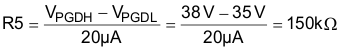Equation 40.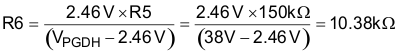Nearest available 1% resistors should be chosen. Set R5 = 150 kΩ and R6 = 10.5 kΩ.

#### 9.2.1.2.8 Input and Output Protection

Proper operation of the LM5066 hot swap circuit requires a voltage clamping element present on the supply side of the connector into which the hot swap circuit is plugged in. A TVS is ideal, as depicted in . The TVS is necessary to absorb the voltage transient generated whenever the hot swap circuit shuts off the load current. This effect is the most severe during a hot-short when a large current is suddenly interrupted when the FET shutts off. The TVS should be chosen to have minimal leakage current at VIN,MAX and to clamp the voltage to under 100V during hot-short events. For many high power applications 5.0SMDJ60A is a good choice.

If the load powered by the LM5066 hot swap circuit has inductive characteristics, a Schottky diode is required across the LM5066’s output, along with some load capacitance. The capacitance and the diode are necessary to limit the negative excursion at the OUT pin when the load current is shut off.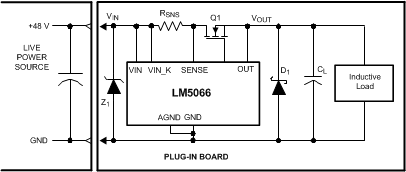Figure 36. Output Diode Required for Inductive Loads

#### 9.2.1.2.9 Final Schematic and Component Values

Figure 28 shows the schematic used to implement the requirements described in the previous section. In addition, Table 47 provides the final component values that were used to meet the design requirements for a 48-V, 10-A hotswap design. The application curves in the next section are based on these component values.

### Table 47. Final Component Values (48-V, 10-A Design)

COMPONENT VALUE
RSNS 2 mΩ
R1 150 kΩ
R2 11.5 kΩ
R3 95.3 kΩ
R4 3.74 kΩ
R5 150 kΩ
R6 10.5 kΩ
RPWR 21 kΩ
Q1 PSMN4R8-100BSEJ
Q2 MMBT3904
D1 B380-13-F
Z1 5.0SMDJ60A
CTIMER 150 nF
Optional dv/dt circuit DNP

#### 9.2.1.3 Application Curves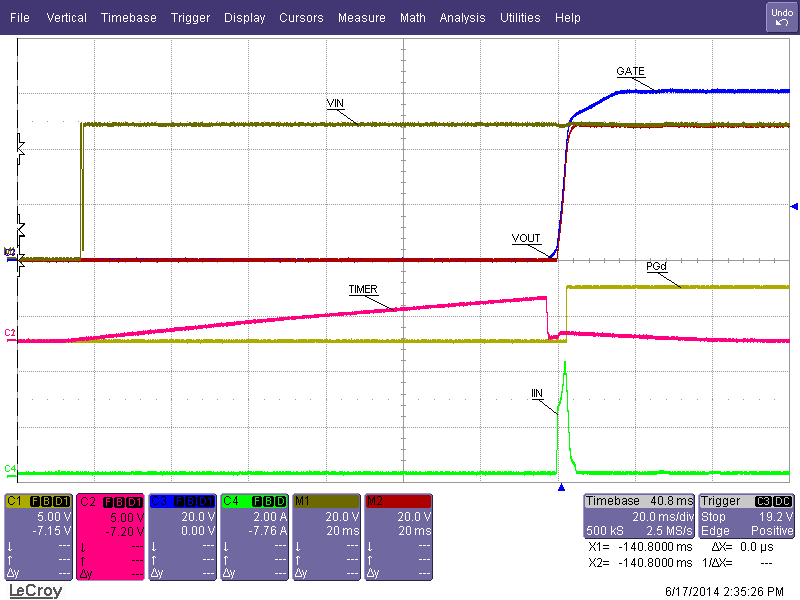Figure 37. Insertion Delay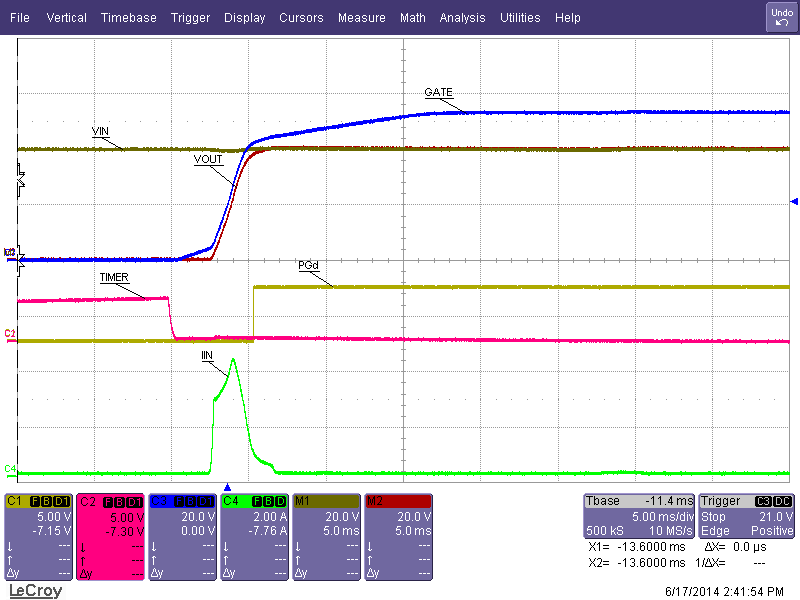VIN = 40V
Figure 39. Start-Up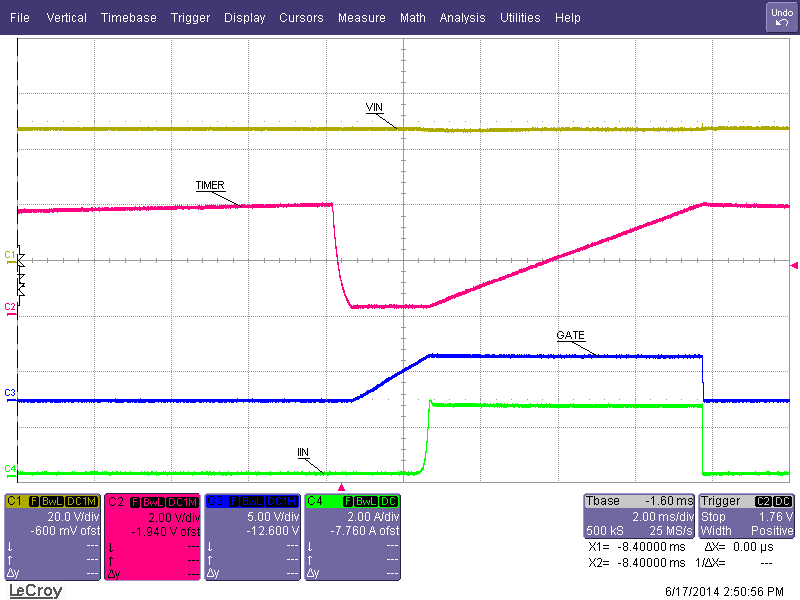Figure 41. Start-Up into Short Circuit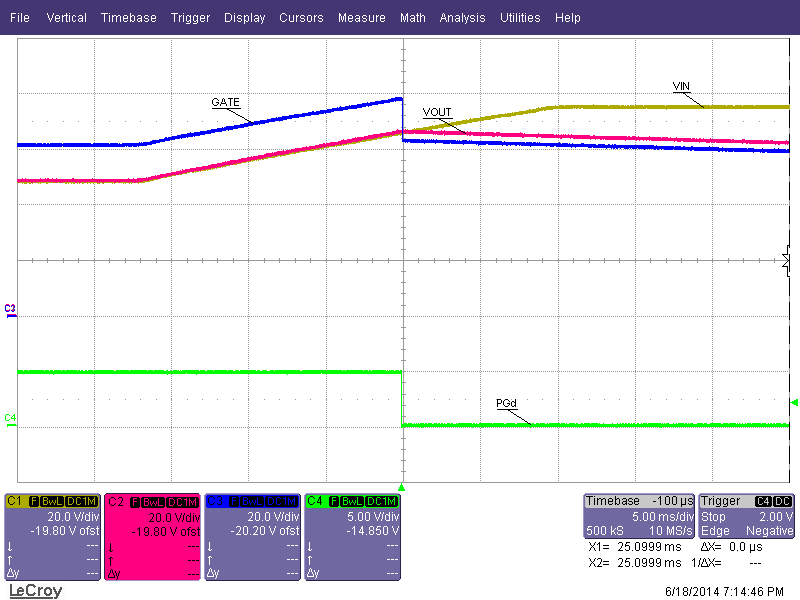Figure 43. Over-Voltage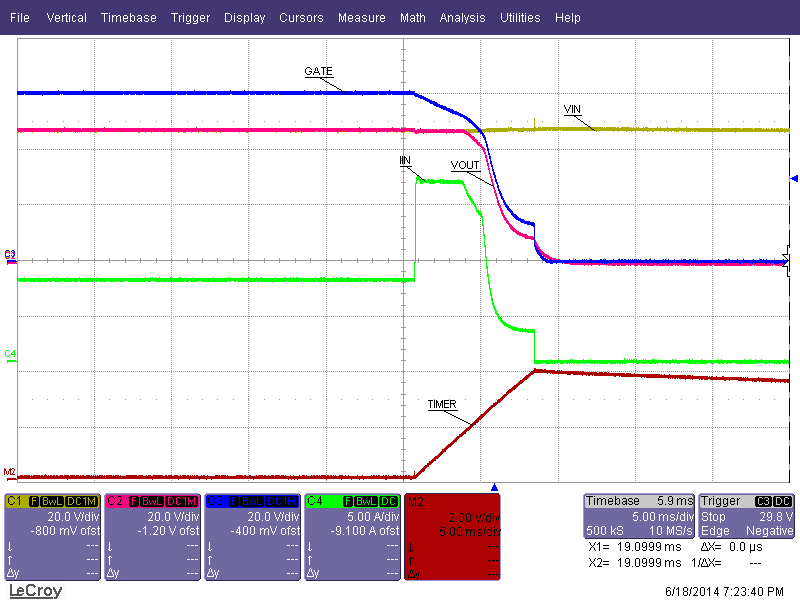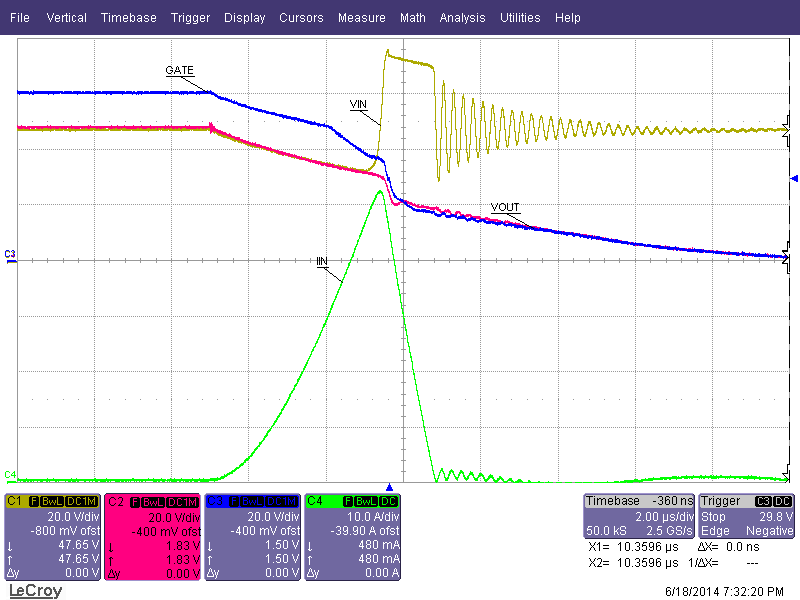Figure 47. Hotshort on Output (Zoomed In)VIN = 48V
Figure 38. Start-Up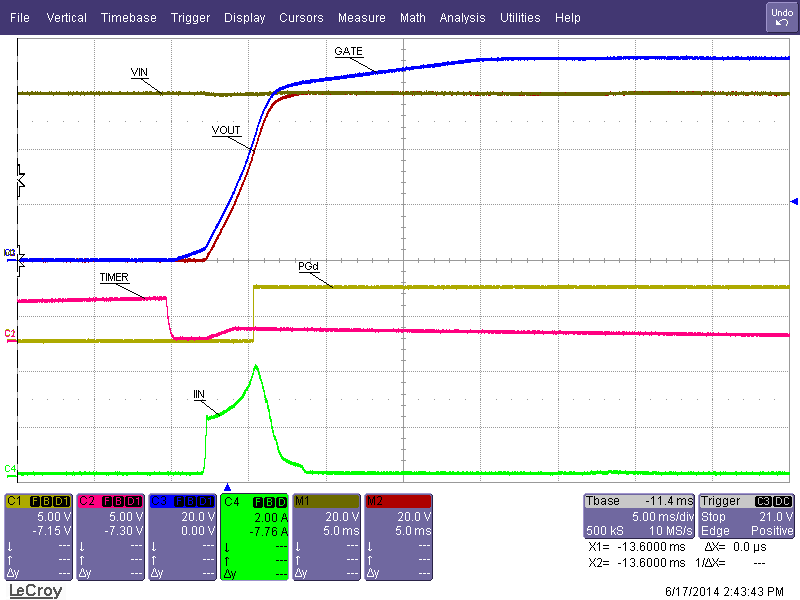VIN = 60V
Figure 40. Start-Up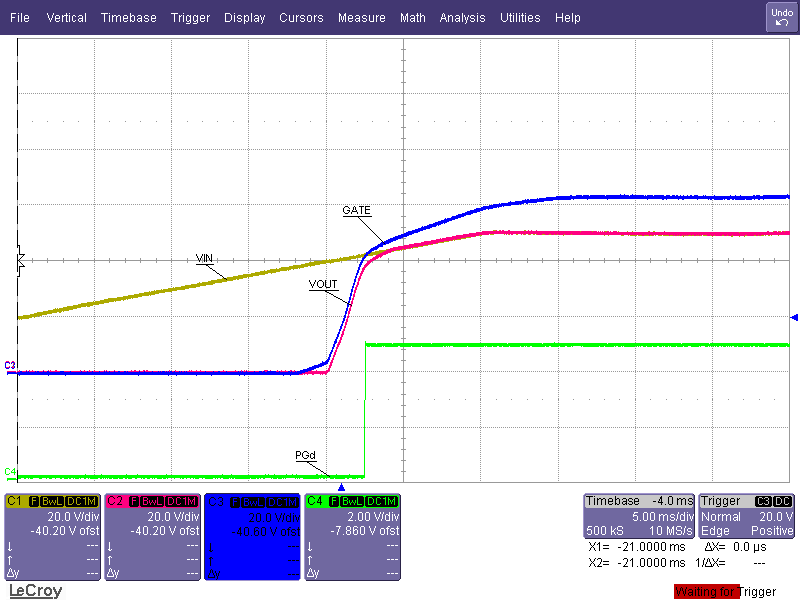Figure 42. Under-Voltage